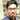# Bit-'Wise' Magic

July 10, 2011

‘Bitwise operators’, as the name says they are indeed wise enough to do anything that a computer can do. In computer architecture, we would have studied how these ANDs, ORs and SHIFTs are used to do any mathematical operation. Any basic arithmetic operation is a set of bitwise operation, which for convenience we have abstracted to a level that we forgot what is underlying. Sometimes, a lower level of abstraction is a better tradeoff to higher level of abstraction; an example is that programs written in assembly language are more efficient than those written in high level language. So we have to pierce through the abstraction to find the right things when we really need right things. Well, I think it is time to learn some bitwise tricks and improve our programming. Presence of bitwise operators in your programs identifies yourself as a quality programmer from my point.

Bitwise operators caught my eyes to write about it, just because it’s very beautiful but many don’t understand its beauty. I had like to bring it to limelight. When I start to write about it, I think it deserves to be a separate book itself; that much of secrets hidden in bitwise operators.

Like all the books I don’t want to give the tables of AND, OR, XOR, NOT, etc because I believe they are ‘alphabets’ if programming is ‘language’. I want to express about the ‘language’ instead of teaching ‘alphabets’. As far as I believe, each and every computer science engineer should be very strong in digital electronics.

It is time to see some bitwise magic.

You can face an interview question that you have to write a program to multiply a number by 4 without using ‘*’ operator. That’s quite simple if you know shift operators really.

``````int a=5;
printf(“%d”,a<<1);``````

The output is 10. a<<1 is equivalent to a*2. How? Let’s see…

Say a is 0000 0101. Left shift by one moves all bits one position left, discarding the leftmost bit and adding a 0 at the rightmost end. a<<1 is 0000 1010.

a = 5 = 0000 0101 = 20+22

Now we left-shift by 1,

a<<1 = 0000 1010 = 21+23 = 2(20+22) = 2(a) = 10

a<<2 = (a<<1)<<1 = (2(a))2 = 22a

The common fact is that a<<n = 2n(a)

Now try to answer the interview question that I first discussed about. It’s simple, shift the number to be multiplied by 4 by to the left by two. That is, N<<2 if the number to multiplied is N.

Another fact is that the reverse is also true… If you right shift by 1, it is equivalent to dividing the number by 2.

i.e., a>>n = a/2n

Now multiply a number N by eight means

N<<3

Divide the same number by 16

N>>4

So now, I believe you have learnt to write a program to multiply or divide a number by a power of two. This is just the beginning of what bitwise can do, I will try to write more further, but I need your comments about everything, from the topic to even the presentation style to improve my writings.Written by Fareez Ahamed who lives and works in Chennai, India building useful things. You should follow him on Twitter